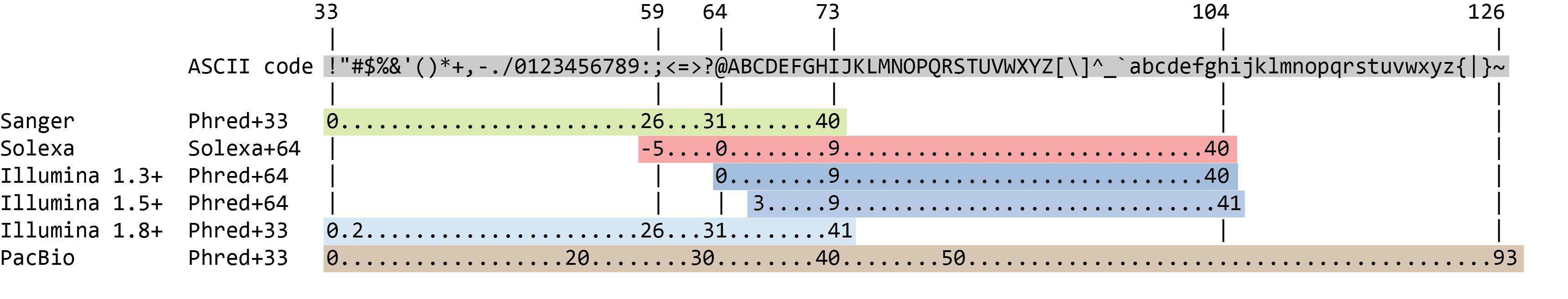### Format

#### FASTQ format

Although it looks complicated (and maybe it is), the FASTQ format is easy to understand with a little decoding. Each read, representing a fragment of DNA, is encoded by 4 lines:

Line Description
1 Always begins with @ followed by the information about the read
2 The actual nucleic sequence
3 Always begins with a + and contains sometimes the same info in line 1
4 Has a string of characters which represent the quality scores associated with each base of the nucleic sequence; must have the same number of characters as line 2

So for example, the first sequence in our file is:

@03dd2268-71ef-4635-8bce-a42a0439ba9a runid=8711537cc800b6622b9d76d9483ecb373c6544e5 read=252 ch=179 start_time=2019-12-08T11:54:28Z flow_cell_id=FAL10820 protocol_group_id=la_trappe sample_id=08_12_2019AGTAAGTAGCGAACCGGTTTCGTTTGGGTGTTTAACCGTTTTCGCATTTATCGTGAAACGCTTTCGCGTTTTCGTGCGGAAGGCGCTTCACCCAGGGCCTCTCATGCTTTGTCTTCCTGTTTATTCAGGATCGCCCAAAGCGAGAATCATACCACTAGACCACACGCCCGAATTATTGTTGCGTTAATAAGAAAAGCAAATATTTAAGATAGGAAGTGATTAAAGGGAATCTTCTACCAACAATATCCATTCAAATTCAGGCA+$'())#$$%#%%'-&%'%#%('+;<>>>18.?ACLJM7E:CFIMK<=@0/.4<9<&007:,3<IIN<3%+&(+#%'#.2@401/5=49IEE=CH.20355>-@AC@:B?7;=C4419)*$$46211075.$%..#,529,''=CFF@:<?9B522.(&%%(9:3E99<BIL?:>RB--**5,3(/.-8B>F@@=?,9'36;:87+/19BAD@=8*''&''7752'$%&,5)AM<99$%;EE;BD:=9<@=9+%$ It means that the fragment named @03dd2268-71ef-4635-8bce-a42a0439ba9a (ID given in line1) corresponds to: • the DNA sequence AGTAAGTAGCGAACCGGTTTCGTTTGGGTGTTTAACCGTTTTCGCATTTATCGTGAAACGCTTTCGCGTTTTCGTGCGGAAGGCGCTTCACCCAGGGCCTCTCATGCTTTGTCTTCCTGTTTATTCAGGATCGCCCAAAGCGAGAATCATACCACTAGACCACACGCCCGAATTATTGTTGCGTTAATAAGAAAAGCAAATATTTAAGATAGGAAGTGATTAAAGGGAATCTTCTACCAACAATATCCATTCAAATTCAGGCA (line2) • this sequence has been sequenced with a quality $'())#$$%#%%'-&%'%#%('+;<>>>18.?ACLJM7E:CFIMK<=@0/.4<9<&007:,3<IIN<3%+&(+#%'#.2@401/5=49IEE=CH.20355>-@AC@:B?7;=C4419)*$$46211075.$%..#,529,''=CFF@:<?9B522.(&%%(9:3E99<BIL?:>RB--**5,3(/.-8B>F@@=?,9'36;:87+/19BAD@=8*''&''7752'$%&,5)AM<99$%;EE;BD:=9<@=9+%$ (line 4).

But what does this quality score mean?

The quality score for each sequence is a string of characters, one for each base of the nucleotide sequence, used to characterize the probability of misidentification of each base. The score is encoded using the ASCII character table (with some historical differences):So there is an ASCII character associated with each nucleotide, representing its Phred quality score, the probability of an incorrect base call:

Phred Quality Score Probability of incorrect base call Base call accuracy
10 1 in 10 90%
20 1 in 100 99%
30 1 in 1000 99.9%
40 1 in 10,000 99.99%
50 1 in 100,000 99.999%
60 1 in 1,000,000 99.9999%

#### Quality Scores

But what does this quality score mean?

The quality score for each sequence is a string of characters, one for each base of the nucleotide sequence, used to characterize the probability of misidentification of each base. The score is encoded using the ASCII character table (with some historical differences):

To save space, the sequencer records an ASCII character to represent scores 0-42. For example 10 corresponds to “+” and 40 corresponds to “I”. FastQC knows how to translate this. This is often called “Phred” scoring.So there is an ASCII character associated with each nucleotide, representing its Phred quality score, the probability of an incorrect base call:

Phred Quality Score Probability of incorrect base call Base call accuracy
10 1 in 10 90%
20 1 in 100 99%
30 1 in 1000 99.9%
40 1 in 10,000 99.99%
50 1 in 100,000 99.999%
60 1 in 1,000,000 99.9999%

What does 0-42 represent? These numbers, when plugged into a formula, tell us the probability of an error for that base. This is the formula, where Q is our quality score (0-42) and P is the probability of an error:

Q = -10 log10(P)

Using this formula, we can calculate that a quality score of 40 means only 0.00010 probability of an error!

### Visualisation

#### Using IGV with Galaxy

You can send data from your Galaxy history to IGV for viewing as follows:

• In IGV, go to View > Preferences > Advanced
• Check the box Enable Port
• Under display in IGV, click on local